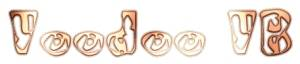Direct3D Rotation

 Wierdo (2/18/00 4:58:01 am) Hi, I'm new at Direct3d and I got a problem with rotating polygons. I can rotate it in one axis but not two or three at the same time. If i write that it should rotate in x and then y, then it only rotats around the y axis.    Can someone set up some code or just explain what I'm doing wrong. thanks,   Daniel Netz (2/18/00 8:02:43 am) I dunno since I haven't had time to get into transformations yet, but you should check the D3DIM samples out from the DX7 SDK, I'm sure you can find rotation codes there. Daniel Netz, Sentinel Design   Corre (2/18/00 3:20:54 pm) Here is what I do, and this works for me: Dim WorldMatrix as D3DMATRIX Dim Temp As D3DMATRIX 'Temp matrix to hold rotations Dim Rot As D3DMATRIX 'Temp rotation matrix 'Modify WorldMatrix to create a translation matrix DirectX.IdentityMatrix WorldMatrix WorldMatrix.rc41 = X WorldMatrix.rc42 = Y WorldMatrix.rc43 = Z DirectX.IdentityMatrix Rot 'Sets up the rotation matrix 'Using the left-to right order of matrix concatenation, 'apply the translation to the object's world position 'before applying the rotations. 'First, pitch If Pitch <> 0 Then DirectX.RotateXMatrix Temp, Pitch DirectX.MatrixMultiply Rot, Rot, Temp End If 'Then, yaw If Yaw <> 0 Then DirectX.RotateYMatrix Temp, Yaw DirectX.MatrixMultiply Rot, Rot, Temp End If 'Finally, roll If Roll <> 0 Then DirectX.RotateZMatrix Temp, Roll DirectX.MatrixMultiply Rot, Rot, Temp End If 'Apply the rotation matrices to the translation already in 'WorldMatrix to complete the world matrix DirectX.MatrixMultiply WorldMatrix, Rot, WorldMatrix Device3D.SetTransform D3DTRANSFORMSTATE_WORLD,WorldMatrix   In this example X, Y and Z holds the position of the object, and Roll, Picth and Yaw holds the orientation hope this helps /Corre, MiCo Games micogames.cjb.net